# Non Verbal Reasoning - Cubes and Dice

### Exercise :: Cubes and Dice - Section 1

The sheet of paper shown in the figure (X) given on the left hand side, in each problem, is folded to form a box. Choose from amongst the alternatives (1), (2), (3) and (4), the boxes that are similar to the box that will be formed.

6.

Choose the box that is similar to the box formed from the given sheet of paper (X).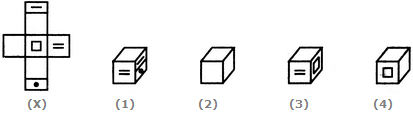A. 1 only B. 1 and 3 only C. 1, 3 and 4 only D. 1, 2, 3 and 4

Explanation:

When the sheet in fig. (X) is folded, then one of the faces of the cube formed will be of the form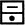and this face will lie opposite the face bearing a square. Also, one of the blank faces lies opposite another blank face and the third blank face lies opposite the face bearing an '=' sign. Clearly, all the three blank faces cannot appear adjacent to each other. So, the cube shown in fig. (2) which has all the three blank faces adjacent to each other cannot be formed. Hence, only the cubes shown in figures A, C and D can be formed.

7.

Choose the box that is similar to the box formed from the given sheet of paper (X).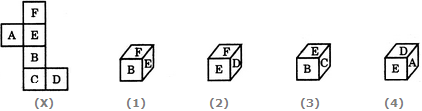A. 1 only B. 2 only C. 1 and 3 only D. 1, 2, 3 and 4 only

Explanation:

The fig. (X) is similar to the Form III. So, when the sheet in fig. (X) is folded to form a cube, then 'F' appears opposite 'B', 'E' appears opposite 'C' and 'A' appears opposite 'D' Therefore, the cube in fig. (1) which shows 'F' adjacent to 'B' the cube in fig. (3) which shows 'E' adjacent to 'C' and the cube in fig. (4) which shows 'A' adjacent to 'D' cannot be formed. Hence, only the cube in fig.(2) can be formed.

8.

Choose the box that is similar to the box formed from the given sheet of paper (X).A. 1 only B. 2 only C. 3 only D. 4 only

Explanation:

The fig. (X) is similar to the Form III. So, when the sheet in fig. (X) is folded to form a cube, then the half-shaded face appears opposite to the face bearing a rhombus, the face with a black circle appears opposite to one of the two blank faces and the face with a '+' sign appears opposite to the other blank face. Clearly, the cubes shown in figures (1) and (4) cannot be formed since they have the half-shaded face adjacent to the face bearing the rhombus. Also, though the cube shown in fig. (3) has faces that can appear adjacent to each other but the cube formed by folding the sheet in fig. (X) cannot be rotated to form fig. (3). Hence, the cube in fig. (3) cannot be formed. Thus, only the cube shown in fig. (2) can be formed.

9.

Choose the box that is similar to the box formed from the given sheet of paper (X).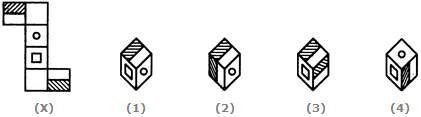A. 1 only B. 2 only C. 3 only D. 4 only

Explanation:

The fig. (X) is similar to the Form II. So, when the sheet shown in fig. (X) is folded to form a cube then the two half-shaded faces lie opposite to each other, the face bearing a square lies opposite to one of the two blank faces and the face bearing a circle lies opposite to the other blank face. Therefore, the cubes shown in figures (2) and (3) which have the two half-shaded faces adjacent to each other, cannot be formed by folding the sheet shown in fig. (X). Also, though the cube shown in fig. (4) has faces that can appear adjacent to each other but the cube formed by folding the sheet in fig. (X) cannot be rotated to form the cube in fig. (4). Hence, only the cube in fig. (1) can be formed.

10.

Choose the box that is similar to the box formed from the given sheet of paper (X).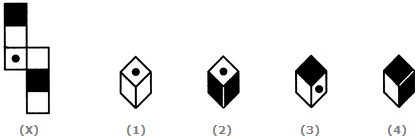A. 2 and 3 only B. 1, 3 and 4 only C. 2 and 4 only D. 1 and 4 only